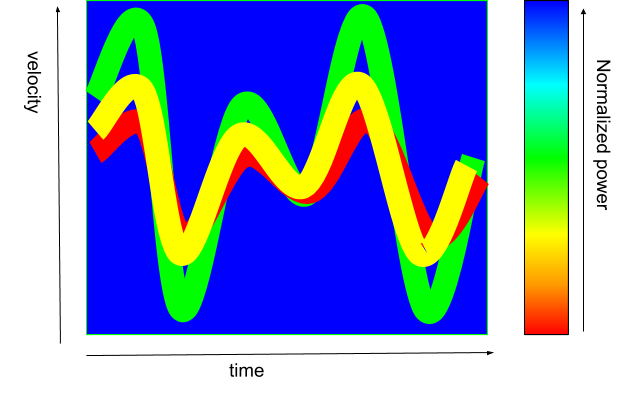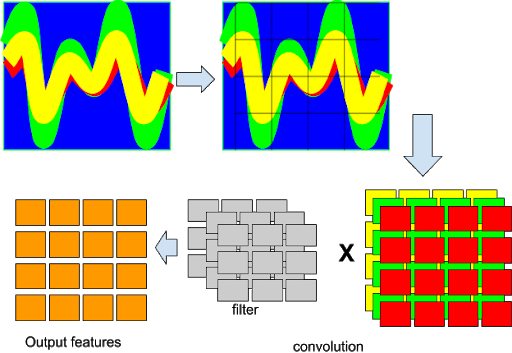•written on Dec 26, 2019
by Martin Rupp

Artificial Intelligence - A Short Overview of Classification Techniques for RADAR Targets through Neural Networks 2/2A relevant technique to classify RADAR object is to use a colored 2D map representing range, speed or frequency against time and color the map with power intensity. Here we represent examples of such typical RADAR data.

In the first article of this series, we presented an overview of the techniques used for classification with the neural networks. Here we dive into an example.Once that representation has been generated, it can be processed as an image. Automatic recognition of images by neural networks is very advanced and mostly often uses convolutional neural networks. The same process is generally used for automatic recognition of RADAR data.

Convolutional Neural Networks

Convolutional Neural Networks (CNNs) are a special class of neural networks generalizing multilayer perceptrons (eg feed-forward networks ). CNNs are primarily based on convolution operations, eg ‘dot products’ between data represented as a matrix and a filter also represented as a matrix. The Convolution operation can be seen as an alternative to the Matrix product. The result can be seen as a regularization or ‘smoothing’ of the raw input data.

A typical Convolutional network will create a 3D matrix from RADAR data. For example the previous RADAR sampled data will be represented as a matrix of velocity x time x power intensity.In the above representation, each cell represents a neuron.

This technique involves spatial data which are invariant under translation shifts. For this reasons, convolutional neural networks are also called shift invariant or space invariant artificial neural networks (SIANN).

The convolution layer is usually followed by a pooling layer and that is repeated for as many rounds as necessary,

Typical operations performed by the neural networks to classify RADAR data

Here we list and detail the main operations performed by a typical neural network used to classify RADAR data.

 operation definition convolution A “filter” is applied over the RADAR data (image),the filter scans a range of “cells”at a time and it builds an output feature matrix which is able to predict the class to which each of the feature belongs pooling Pooling is also the practice of downsampling. It reduces the amount of information after convolution has been applied. There are different types of pooling: max-pooling or average pooling for instance. The idea is to find the maximum/average value in a “pool” of data inside the values and replace that pool by that unique value. subsampling Normalize all data in a pool by giving all of them a unique value. It’s similar to pooling. dropout A technique to prevent overfitting which happens when the network is fully connected.Dropout will set some weights to zero and concretely will remove some neurons and connections from the network, usually randomly. flatten Reduce the dimensions of an input layer dense Totally connects one layer to another softmax Maps the output to a normalized probability distribution representing the probability of appearance in a class.

Some classical neural network designs for RADAR classification

Over the years, several designs have been found to behave optimally for several classes of problems, usually Image Recognition. Here we list several neural networks designs that have been successfully used for RADAR data classification.

Lenet-5

The LeNet architecture is one of the first CNN and was developed by Yann LeCun, Leon Bottou, Yoshua Bengio and Patrick Haffner in 1998. This design became very popular for the automatic recognition of handwritten digits and for document recognition in general. LeNet-5 was successfully used in order to achieve the classification of hand-written digits on bank cheques in the U.S.A.

LeNet-5 has 6 hidden layers C1→S2→C3→S4→C5→F6.

C1, C3, C5  are convolution layers.S1, S4  are pooling layers and the last layer, F6, achieves fully connection.

This is a relatively simple design, at least from a modern perspective. It has some unusual features. For instance not all the data produced by the layer S2 are used by the following layer, the convolution layer C3 so to produce different patterns from different outputs.

The LeNet designs are multilevel machine learning algorithm using spatial relationships so to reduce the amount of parameters and therefore improving training performance

LeNet-5 model was successfully used in   to classify moving targets from RADAR Doppler data reflecting the changes in velocity (e.g acceleration/.deceleration) . The model was used as an image classificator from time-frequency image representations.

VGGNet

VGGNet is a ‘modern’ CNN which consists of 16 convolutional layers. It bears similarities to the AlexNet but have more filters. VGGNET has 1.38 million parameters and so it consumes a lot of CPU power.

Designs inspired from VGGNET were applied in the context of RADAR classification in  and . It successfully distinguished objects obtained from RADARs placed in automotive systems. In  it could classify objects scanned by a car front RADAR and automatically decide if they were pedestrians, cyclists or other cars.

The GoogleNet design is based on a 22 layer deep CNN using a sub-module which performs small convolutions and which is called by the designers “inception

Module”. This inception module uses batch normalization and the gradient descent method known as RMSprop. The number of parameters are around 4 millions and it also requires important CPU power to be run.

GoogleNet was applied in  and  to classify RADAR data. In  the neural net was applied to imagery data representing frequency over time. The time-frequency image was generated after CWD (Choi-Williams distribution) transformation of 8 LPI (Low probability of intercept) radar signals.

AlexNet

AlexNet is based on five convolutional layers which are followed by three fully connected layers. It also uses very extensively the ReLu activation function. In the context of RADAR classification it was applied in . AlexNet has more than 60 millions of parameters and so it is one of the most powerful CNN design.

ResNet

The resNet family includes designs named ResNet50 or ResNet18. Resnets are residual neural networks which use the concept of pyramidal cells similar to the ones found in biological cortex neurons. ResNets use skips to jump from one layer to another ‘distant’ layers so to avoid the problem of vanishing gradients. A typical residual network such as ResNet50 is 50 layers deep. It was used in  and . In  ResNets could classify data from Compact polarimetric synthetic aperture radar (CP SAR) and decide the nature of a ship.

Other designs

Such neural networks designs like DivNet-15, ZFnets, Densenets and U-nets have been also applied in the context of RADAR classification. It is too early to decide which design fits best to a RADAR problem since contrary to image classification, using neural networks for RADAR data is just starting

Stay tuned!